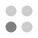• 7,703 trophies earned
• 376 Players Tracked
• 21 Total trophies
• 209 Obtainable EXP
• 1,170 Points
• 0 Platinum Club
• 361 100% Club

Energy Cycle Edge trophies

•Good job! Completed all tasks!96.54%
•Solved first puzzle100.00%
•Puzzles 2, 3 and 4 solved98.67%
•Puzzles 5, 6 and 7 solved98.40%
•Puzzles 8, 9 and 10 solved98.40%
•Puzzles 11, 12 and 13 solved97.87%
•Puzzles 14, 15 and 16 solved97.61%
•Puzzles 17, 18 and 19 solved97.34%
•Puzzles 20, 21 and 22 solved97.07%
•Puzzles 23, 24 and 25 solved97.07%
•Puzzles 26, 27 and 28 solved97.07%
•Puzzles 29, 30 and 31 solved97.07%
•Puzzles 32, 33 and 34 solved96.81%
•Puzzles 35 and 36 solved96.81%
•Puzzles 37, 38 and 39 solved96.81%
•Puzzles 40 and 41 solved96.81%
•Puzzles 42 and 43 solved96.81%
•All of puzzles solved96.54%
•Took less than 10 seconds to solve the puzzle98.67%
•Took more than 15 minutes to solve the puzzle97.34%
•3D puzzle is solved exactly with one rotation98.40%